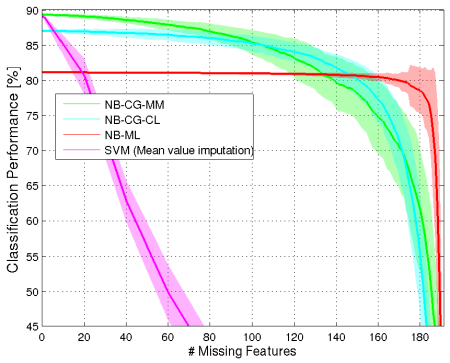Signal Processing and Speech Communication Laboratory

Maximum Margin Bayesian Network Classifiers

Published
Mon, Aug 01, 2011
Tags
rotm
ContactClassification is an important task in machine learning. It deals with assigning a given object to one of a number of different categories. We present a maximum margin parameter learning algorithm for Bayesian network classifiers using a conjugate gradient method for optimization to solve this task. In contrast to previous approaches, we maintain the normalization constraints of the parameters of the Bayesian network during optimization, i.e. the probabilistic interpretation of the model is not lost. This enables to handle missing features in discriminatively optimized Bayesian networks. The potentials of the proposed method as well as a comparison to other existing work on maximum margin Bayesian networks is focus of this work.

The above figure illustrates the capability of the presented maximum margin Bayesian network classifiers in dealing with missing features in the Washington D.C. Mall dataset (details on this dataset can be found in the publication). It shows the classification performance of different classifiers over a varying number of missing features. NB-CG-MM refers to a naive Bayes classifier with maximum margin probability parameters obtained by applying the proposed algorithm, NB-CG-CL is a naive Bayes classifier with probability parameters trained to maximize the conditional likelihood. NB-ML is a naive Bayes classifier with maximum likelihood parameters and SVM (Mean value imputation) refers to classification using a support vector machine where missing features were completed using mean value imputation techniques.